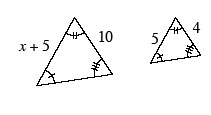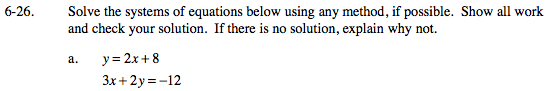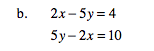Home > GC > Chapter 6 > Lesson 6.1.3 > Problem6-26

6-26.
1. Solve the systems of equations below using any method, if possible. Show all work and check your solution. If there is no solution, explain why not. Homework Help ✎

1. y = 2x + 8

3x + 2y = −12

2. 2x − 5y = 4

5y − 2x = 10Use the substitution method. Remember to check your answer.

x = −4, y = 0Use the Elimination Method.

2x − 5y = 4
−2x + 5y = 10
0 + 0 = 14

0 ≠ 14

No solution, the lines are parallel.